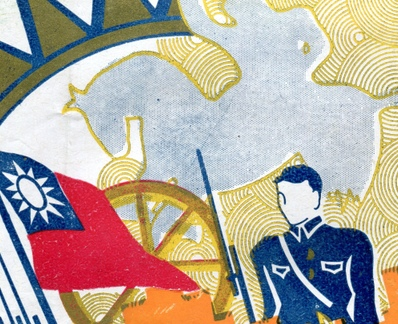•  注 册

中央陆军军官学校第十二期广州分校学生总队同学录2021-07-04 20:04:45  来源：静思斋 于岳  点击：说明：本总队毕业于1938年，以下同学除工兵队叶嵩之外均有照片，静思斋可制作此同学录影印本。

如有下列黄埔同学后裔需要先辈照片，请与抗日战争纪念网联系。电话：0731-85531328、15116420702 ; QQ:2652168198;微信号：kz15116420702;邮箱：tougao@krzzjn.com;地址：湖南省长沙市岳麓区英才园1片17栋。

步兵大队第一队：99人

李学文(广东惠阳) 钟介明(广东五华) 叶君言(广东梅县) 冯吉常(广东东莞)

李汝光(广东东莞) 裴遐昌(贵州绥阳) 唐良仕(广东恩平) 邬春荣(广东番禺)

余国熙(贵州贵阳) 游深洪(广东东莞) 钟恒懋(广东防城) 黎国熹(广东高要)

刘克英(广东茂名) 黄吉平(广东防城) 陈育宏(广东兴宁) 刘鉴泉(广东东莞)

周兆棠(广东茂名) 彭智交(广东钦县) 李琳珍(广东梅县) 梁翰华(广东高要)

范京曰(广东梅县) 陈德芳(广西北流) 吴持正(广东定安) 李锡波(广东惠阳)

翁正滨(广西灵山) 阮伟英(广东番禺) 叶元盛(广东定安) 邓励兴(广东钦县)

林明芳(广西博白) 张瑞川(广东钦县) 张贤碧(广东五华) 萧发安(贵州黔西)

凌云涛(广东和平) 温登亮(广东防城) 刘辅义(湖南醴陵) 刘子溪(贵州石阡)

郭缵三(广东英德) 方富璟(广东钦县) 李汉忠(广东乳源) 唐俊贤(广西玉林)

黄星平(广东防城) 冉隆直(贵州遵义) 江无畏(广东南海) 何增芳(贵州修文)

黎伯伟(广西梧州) 林格物(广东丰顺) 黄安荣(广东钦县) 陈华义(广东钦县)

李锦文(广东兴宁) 陈焕发(广东兴宁) 刘克和(广东茂名) 蒙显庭(广西横县)

吴鼎铮(广东合浦) 曹家儒(贵州平坝) 魏祖章(广东五华) 林家学(广东合浦)

何家球(广西藤县) 郑大培(广东佛冈) 马瑛(广东番禺) 骆希文(广东龙川)

冯树丰(广东钦县) 林世昌(福建永春) 施应朝(广东阳江) 王贵贤(贵州安顺)

冯建祯(广东番禺) 李锡钊(广东惠阳) 张德辉(福建永定) 冯艺仁(广东中山)

何吉修(广东大埔) 黄永为(广东防城) 吴膺枢(广东汕头) 何志洁(广东合浦)

黄宝秋(广东南海) 陈固(广东蕉岭) 黄元震(广东钦县) 毕朝应(广东花县)

魏柏株(广东五华) 傅健中(广东兴宁) 区作镛(贵州贵阳) 陆子才(广东钦县)

李志(广东蕉岭) 陈肇明(广东合浦) 吕天瑞(广西陆川) 林作屏(贵州思南)

赵康民(广东钦县) 温霖文(广东梅县) 李美璧(广东临高) 傅作舟(广东从化)

应隐贵(贵州修文) 谭英汉(广东阳江) 陈德宏(广东兴宁) 余昭寰(广东五华)

胡宜士(广东防城) 谢子滔(广东高明) 周益群(广东五华) 叶伟浩(广东惠阳)

林志华(广西藤县) 范公廉(湖南邵阳) 郑敬融(广东化县)

备注：照片页翁正滨记载为翁正宾

步兵大队第二队：98人

林裕贵(广东茂名) 黄伯权(广东防城) 黄礼华(广东钦县) 陆宝铨(广东信宜)

陆儒椿(广东防城) 李东成(广东惠阳) 吴肇堂(广东合浦) 石业松(广东合浦)

但功鼎(湖北蒲圻) 刘勉之(广东文昌) 韦珍(广东防城) 戴星昌(贵州遵义)

钟应兴(广东梅县) 张亮兴(广东梅县) 魏善成(广东五华) 周希道(湖南醴陵)

吴先淮(广西玉林) 张煜(广东东莞) 李炳枢(广东丰顺) 莫树芬(贵州荔波)

李宗远(广东丰顺) 胡浚(广东琼东) 湛叶亿(广东增城) 陈广隆(广东信宜)

杨景荣(广东化县) 韦为民(广西武宣) 张诚业(广东合浦) 杨振邦(贵州都匀)

江荣森(广东茂名) 马致中(贵州贵阳) 郝存恕(贵州修文) 李俊山(广东合浦)

许定南(广东儋县) 张光乾(广东潮安) 邹秉揆(广东防城) 萧兆麟(广东中山)

方宣昌(广东钦县) 邓伟丹(广东五华) 徐福臻(广东惠阳) 张声吟(广西博白)

林一青(广东兴宁) 李济中(广东兴宁) 陈喜平(广东潮阳) 容达英(广东崖县)

陈绍(广东新兴) 赵钦铭(广东钦县) 黄岐光(广东兴宁) 卜彦适(广东合浦)

孙立德(广东揭阳) 曾侨云(广东防城) 李凤超(广东钦县) 林家駪(广东合浦)

黄杰夫(广东五华) 陈伟勋(广东钦县) 令狐荣嘉(贵州桐梓) 毛廷彦(贵州余庆)

朱杨英(广东惠来) 黄伟勤(广东五华) 林茂青(广东信宜) 卢仁山(广东崖县)

李永年(广东东莞) 李登廉(广东合浦) 梁景灿(广东信宜) 郑诗忠(广东揭阳)

邓檀祥(广东梅县) 王尚浩(广东钦县) 李钦汉(广东兴宁) 李维扬(广西博白)

孙竹筠(贵州黄平) 黄笼山(湖南湘阴) 刘心潜(湖南武冈) 金结明(广东高明)

闻铭洤(广东钦县) 颜储璧(广东防城) 谢煜田(广东合浦) 潘兆燊(广东钦县)

唐家福(广东合浦) 李肇熙(广东高要) 周永祯(广东东莞) 蔡一民(广东海康)

范荣隆(广东惠阳) 沈朗(广东防城) 申业华(广西容县) 杨鸿青(广东龙川)

廖品璋(广东五华) 梁君义(广东灵山) 王文华(广东琼山) 黄克尧(广东合浦)

李蔚明(广东五华) 梁家铿(广东新会) 徐尚纬(广东防城) 李赵璧(贵州贵阳)

罗恕伯(贵州石阡) 陆儒冠(广东防城) 陈汝驹(广东阳江) 岑国琼(广东澄迈)

黄干华(广东惠阳) 李旦如(广东从化)

备注：照片页黄岐光记载为黄歧光、李赵璧记载为李赵壁

步兵大队第三队：94人

陈华杰(广东灵山) 高哲民(广东梅县) 陈洞明(广东惠来) 周骥良(广西武宣)

黄标(广东灵川) 赖永颐(广东罗定) 潘以贤(广东钦县) 谢作(广东高明)

杜明宗(广东花县) 李长春(广西邕宁) 邓茂棠(广东防城) 吴世宅(广东定安)

李焕良(广东五华) 林耀南(广东合浦) 郭敬林(广东番禺) 侯汉业(广东灵山)

林文駪(广东合浦) 叶列(广东惠阳) 朱绍通(广西平南) 梁茨(广东钦县)

黄禹师(广东钦县) 陈芬(广西苍梧) 石业兴(广东合浦) 林绰韶(广东钦县)

李荣芬(广东防城) 杨海佳(广东合浦) 黄杰生(广东龙川) 刘绮祥(广东兴宁)

唐爵卿(广东防城) 魏任寰(广东五华) 卢晨光(广东梅县) 郭顺儒(广东钦县)

郑可均(广东英德) 黄耀华(广东钦县) 李绵生(广东惠阳) 宋梁材(广东防城)

吕传模(贵州兴义) 何光澧(湖南醴陵) 龙方忠(贵州贵阳) 龙宇兆(广东钦县)

卢福善(江苏上海) 古应天(广东五华) 邱杰凤(广东钦县) 张学文(贵州贵阳)

华振欧(广东始兴) 郭纪初(广东三水) 周贻承(广东合浦) 沈耀梁(广东防城)

谢继藩(湖南醴陵) 黎浩球(广东东莞) 刘惠瞻(广东五华) 胡骏球(广东防城)

丘学儒(广东河源) 廖中辉(广东茂名) 李扬缨(广东顺德) 蔡国藩(广东台山)

林勃菁(广东合浦) 谭锡鋆(广东合浦) 谢玉瑞(广东合浦) 林鸿甲(广东文昌)

黎锡(广东高明) 陈鼎新(广东文昌) 黄启丰(广东防城) 刘家驹(广西桂林)

蓝旺坤(广东梅县) 廖永建(广东海康) 方愈(广东钦县) 李文光(广东梅县)

詹习儒(广东文昌) 刘奇侠(湖南醴陵) 韦国和(广东钦县) 欧家鹤(广东合浦)

彭智文(广东钦县) 李成勋(广东东莞) 李建岩(广东钦县) 符荣芝(广东海康)

吴殿锋(广东防城) 黎永康(广东新会) 符家汉(广东钦县) 黄维睴(广东普宁)

黄伟慎(广东五华) 周祥五(广东崖县) 毛廷锦(广东防城) 陈秀林(广东高明)

罗永遇(广东钦县) 梁伯群(广东新会) 胡永焱(广东吴川) 陈一荒(广东阳春)

谭肇雄(广东灵山) 许鎏芳(广东东莞) 吴家权(广东阳春) 余国屏(贵州遵义)

陈祥(广东高明) 赖海泉(广东灵山)

备注：照片页林绰韶记载为林倬韶

炮兵大队第一队：140人

黄开榆(广东钦县) 周仁(广东南海) 伍荫斌(广东兴宁) 钟国辉(广东梅县)

李春华(广东琼山) 郭景略(广东澄迈) 陈履初(广东新会) 丘德强(广东曲江)

李文标(广东高明) 张上珍(广东丰顺) 赖荣福(广东南雄) 朱荣春(广东文昌)

官沛华(广东始兴) 梁禹东(广东防城) 梁潮波(广东开平) 何家琚(广东东莞)

杨维慧(广东惠阳) 徐昶稜(广东蕉岭) 徐炳麟(广东龙川) 林光谟(广东乐会)

萧冰魂(广东东莞) 顾宝璋(广东新兴) 凌汉鎏(广东番禺) 钟恩浩(广东五华)

丘仁汉(广东平远) 官其彪(广东始兴) 周麟春(湖南澧县) 黄昆朝(广东灵山)

谢擎亮(广东广宁) 郑诗瑞(广东揭阳) 虞远谋(广东连山) 余近仁(广东梅县)

吴先荫(广东连县) 刘辛甫(广东揭阳) 钟有令(广东紫金) 赵善芳(广东钦县)

陈权(广东新丰) 罗赓宗(广东东莞) 容定华(广东灵山) 张正国(贵州遵义)

满荣(广东合浦) 黄桂垣(广东四会) 许兆驺(广东阳江) 谢维澄(广东阳江)

陈敦(广东阳江) 林卓芝(广东中山) 郑秉刚(广东丰顺) 周文辉(广东乐会)

骆亦超(广东花县) 严学游(广东惠阳) 邓传远(广东合浦) 林剌天(广东合浦)

刘秀芬(广东合浦) 梁思伟(广东阳春) 吴东玲(广东恩平) 邓树年(广东阳山)

吴迪南(广东徐闻) 黎维藩(广东合浦) 罗礼廉(广东番禺) 刘礼扬(广东佛冈)

潘逸兴(广东河源) 黄理青(广东钦县) 林铸年(广东花县) 曾能富(广东始兴)

卢春芳(广东梅县) 黄永图(广东化县) 张翕初(贵州黄平) 钟景由(广东茂名)

钟强(广东梅县) 程启祥(广东番禺) 周积基(广东茂名) 余云程(广东饶平)

王文(广东海康) 黄尔宁(广东钦县) 朱佳龙(广东电白) 张之驹(广东番禺)

刘汉平(广东始兴) 黄葆荪(广东蕉岭) 丘世贵(广东儋县) 冼伟智(广东合浦)

邓哲(广东曲江) 周远扬(贵州麻江) 谢子武(广东临高) 顾增爵(广东四会)

蔡克谐(广东万宁) 罗芎香(广东兴宁) 岑政任(贵州定番) 冯倬章(广东钦县)

陈泽樵(广东海康) 王灿干(广东东莞) 林崧南(广东儋县) 陈英昌(广东信宜)

吴邦杰(贵州都匀) 黄墨苍(广东连县) 王国元(广东化县) 邹世良(广东五华)

邹广滨(广东龙门) 王辅英(广东琼山) 陈自騵(广东琼山) 吴桐章(贵州都匀)

陈广图(广东蕉岭) 周轫初(广东澄海) 赵玉光(广东钦县) 陈光鼎(贵州息烽)

张桂芬(广东博罗) 刘达韬(广东佛冈) 何廷垣(广东中山) 玉永标(广东钦县)

甘容武(广东曲江) 黄纲才(广东钦县) 郝德权(广东番禺) 黄金裕(广东惠阳)

马国勋(广东合浦) 黄世杰(广东五华) 蔡若超(广东揭阳) 禤鸿仪(广东曲江)

蔡广泰(广东澄海) 劳善和(广东徐闻) 罗会云(贵州都匀) 江祖安(广东清远)

谭子贤(广东新会) 利锦英(广东新会) 胡善群(贵州麻江) 姚树楷(广东钦县)

罗友仁(广东从化) 黄伟勋(广东蕉岭) 叶国鑫(广东信宜) 梁钦浩(广东高明)

阮平一(广东东莞) 符世香(广东文昌) 黄守范(广东文昌) 李炼琼(广东从化)

张迪乾(广东兴宁) 杨善辉(广东电白) 王殷三(广东琼山) 黄冠球(广东东莞)

曾昭仁(广东始兴) 邓伟权(广东兴宁) 李怀德(广东信宜) 张荣华(广东新会)

炮兵大队第二队：104人

黄志君(广东博罗) 戴诗章(广东阳江) 黄锦沛(广东台山) 邝纪云(广东花县)

张杏芬(广东丰顺) 禤修存(广东防城) 曾育智(广东和平) 陈树芬(广东兴宁)

李谷贤(广东兴宁) 周昭武(广东琼山) 陈少芳(广东兴宁) 李琼璜(广东吴川)

邹治邦(广东南海) 曾智昌(广东潮安) 魏治成(广东五华) 卢连佳(广东徐闻)

郑肇辀(广东高要) 陈慎慧(广东阳江) 许名显(湖南湘阴) 钟文敬(广东新会)

陈大本(广东惠阳) 区宝志(广东云浮) 洪智(广东澄迈) 关仲志(广东番禺)

梁贤温(广东廉江) 陈健贤(广东廉江) 陈燮枢(广东东莞) 林冠民(广东增城)

丘梅荣(广东蕉岭) 陈均和(广东曲江) 李君宁(广东化县) 陈名标(广东揭阳)

谈玉麟(广东中山) 江秦兴(广东廉江) 郭坚(广西苍梧) 萧秉中(广东中山)

邓称康(广东合浦) 王国榜(广东琼山) 黎国韬(广东高要) 任高年(广东鹤山)

张继香(广东龙川) 谢必宽(广东合浦) 曹凯元(广东梅县) 廖家让(广东云浮)

吕道生(广东鹤山) 李益三(广东钦县) 张俊球(广东五华) 茹玉振(广东曲江)

梅建章(广东台山) 钟伯岳(广东花县) 陈翰光(广东从化) 范乃涛(广东阳春)

陈有成(广东德庆) 曹维彬(广东合浦) 何炽成(广东鹤山) 张汉德(广东鹤山)

姚纪昭(广西博白) 朱明熙(广东五华) 古一恒(广东五华) 陈伟亮(广东兴宁)

吴绍夔(广东恩平) 黄和文(广东龙川) 叶若萍(广东惠阳) 王秀儒(广东澄迈)

谢自俊(广东文昌) 梁朝赞(广西藤县) 萧毓仁(广东徐闻) 林焕卿(广东郁南)

赖子扶(广东曲江) 蒋芳兰(广西博白) 黎植榯(广西苍梧) 赖棨鑫(广东钦县)

黎鋆华(广东信宜) 何任廷(广西苍梧) 凌耀中(广东梅县) 王绍涣(广东东莞)

王宝尧(广东合浦) 陈式岩(广东潮阳) 蒋善谦(广东三水) 曾虞书(广东揭阳)

张时度(广东新会) 胡孟刚(广东开平) 魏颂年(广东五华) 陈德惠(广西博白)

许江权(广东新会) 陈郁文(广东东莞) 杨炳明(广东翁源) 龙祖德(广西柳州)

黄嵩高(广东灵山) 方克明(广东开平) 陈卓(广东化县) 陈应鸿(广东海康)

谢耀溪(广东从化) 袁翰海(广东化县) 鲁骏(贵州贵阳) 金文汉(贵州修文)

邓玉树(广东鹤山) 欧阳光(广东花县) 罗德崇(广东合浦) 何芹泮(广东恩平)

黄觉清(广东台山) 吕德风(广东廉江) 袁克夷(广东龙川) 龙怡颜(广东英德)

备注：江秦兴照片页载为江泰兴

工交科工兵队：60人

陈国邦(广东台山) 李宗熀(广东信宜) 刘天培(广东高明) 李增芳(广东廉江)

杨善祥(广东电白) 李业兴(广东钦县) 陈玉春(广东文昌) 黄绍国(广东钦县)

邓兆墉(广东东莞) 王家抟(广东花县) 吴湛成(广东开平) 韩荣辉(广东文昌)

陈文略(广东高明) 莫礼汉(广东鹤山) 章传光(广东钦县) 李彬西(广东钦县)

罗子绰(广东新兴) 甘福民(广东翁源) 李体球(广东钦县) 牟开荣(贵州贵阳)

曾镇锴(广东钦县) 金捷明(广东高明) 周奎(广东钦县) 余觉民(广东台山)

张振华(广东五华) 陈肇芳(广东合浦) 林秀芬(广东合浦) 张植权(广东花县)

邓开诚(广东花县) 陈仲坤(广东五华) 黄国屏(广东廉江) 刘发胜(广东海康)

陈大新(广东澄海) 刘瑞祥(广东河源) 黄慕修(广东钦县) 黎启明(广东钦县)

莫绍华(广东茂名) 张乾鉴(广东灵山) 程佩辉(广东河源) 黄镜波(广东灵山)

冯景萱(广东鹤山) 黄以睗(广东钦县) 叶文杰(广东梅县) 萧昭松(广东平远)

钟贤声(广东廉江) 陈葵荣(广东海康) 张耀球(广东花县) 张国兴(广东五华)

刘昌河(广东花县) 黄之柱(广东钦县) 董之经(贵州镇远) 王持衡(贵州仁怀)

陈萃周(广东翁源) 刘安庸(贵州桐梓) 林绳规(广东信宜) 邝有通(广东花县)

蒙天庥(贵州黔西) 黎德熊(广东阳春) 李润生(贵州都匀) 黎锡杰(广东廉江)

备注：照片页李体球记载为李启球、刘发胜记载为刘法胜、黄以睗记载为黄以旸、黎锡杰记载为黎锦杰

经理科经理队：114人

薛炯棠(广东台山) 黄荫周(广东从化) 郭熹(广东番禺) 梁英(广东海康)

姚育才(广东平远) 黄明铨(广东新兴) 梁鼎章(广东琼山) 梁济时(广东茂名)

梁少元(广东顺德) 古弼(广东中山) 林华(广东蕉岭) 谭耀华(广东开平)

戴天职(广东惠阳) 童绍鼎(广东番禺) 何国桢(广东番禺) 周柏(广东茂名)

叶嵩(广东梅县) 黄鹏程(广东新会) 叶思永(广东封川) 杨家干(广东番禺)

陆士访(广东信宜) 彭*谟(广东连平) 欧绳祖(广东信宜) 徐文煊(广东南海)

陈宝鉴(广东兴宁) 郭德麟(广东蕉岭) 陈福泉(广东平远) 张用之(广东开平)

李天威(广东龙川) 李文棠(广东南海) 钟镇坡(广东东莞) 钟华炬(湖南蓝山)

关秉裕(广东南海) 陈汝谋(广东茂名) 黄伟雄(广东五华) 何传题(广东连县)

罗炎昌(广东南海) 黄尚义(广东台山) 宋性善(广东花县) 杨元辉(广东英德)

敖华裴(广东阳江) 巫远猷(广东兴宁) 王赋才(广东花县) 姚鋈梁(广东茂名)

梁应桓(广东信宜) 唐光祖(广西桂林) 张书铨(广东文昌) 李竞芳(广东信宜)

罗文广(广东惠阳) 赵道修(广东和平) 汤耀民(广东花县) 钟强(广东新会)

陈光德(广东台山) 巫运生(广东梅县) 林杏荣(广东平远) 郑尚贤(广东高要)

何杰(广东南海) 李子仓(广东番禺) 廖树培(广东新会) 潘元坚(广东罗定)

李君隆(广东信宜) 邹敬文(广东惠阳) 欧镒锟(广东顺德) 麦顺庭(广东鹤山)

刘鉴坚(广东番禺) 萧寿千(广东中山) 郑国庚(广东佛冈) 颜惠谋(广东惠阳)

苏宝林(广东合浦) 林贻栋(广东新会) 罗寿山(广东陆丰) 何熙荣(广东德庆)

陈泽驹(广东清远) 谢家槐(广东儋县) 黄逸才(广东高要) 卓荣雄(广东五华)

陈兆东(广东文昌) 苏文彬(广东合浦) 朱家栋(广东合浦) 胡宜懋(广东防城)

黄长璋(广东防城) 黄步先(广东阳江) 梁树德(广东阳江) 萧喜寿(广东梅县)

卢崇惠(广东南海) 苏惠畴(广东惠阳) 廖冠球(广东五华) 唐新财(广东恩平)

陈銮坡(广东新会) 周墀(广东番禺) 李金麟(广东鹤山) 陈有耀(广东遂溪)

彭祖年(广东新兴) 梁同连(广东新会) 陈少泉(广东南海) 梁望俊(广东信宜)

刘达荣(广东中山) 张启瑞(广东东莞) 朱光鉴(广东始兴) 温开信(广东梅县)

孙光复(广东崖县) 周炳文(广东琼山) 詹振球(广东五华) 李霭芬(广东兴宁)

罗正邦(广东电白) 关绪柄(广东崖县) 李伟祥(广东兴宁) 何超群(广东丰顺)

王树藩(广东信宜) 黄传忠(广东潮安) 刘琴生(广东东莞) 叶毓昌(广东惠阳)

林钰桓(广东梅县) 朱信发(广东梅县)

备注：照片页赵道修记载为赖道修、苏宝林记载为苏宝琳、卓荣雄记载为卓乐雄

公交科通信队：85人

刘孟芳(广东五华) 陈镇清(广东罗定) 刘炳钟(广东惠阳) 张瑞衡(广东平远)

李昭礼(广西蒙山) 王宝汉(广东合浦) 李荧(广东惠阳) 李中健(广东化县)

侯秦(广东梅县) 李弃儿(广东兴宁) 李宜春(广东惠阳) 周祖文(广西玉林)

刘华(广东龙川) 张宏俊(广东兴宁) 陈本昌(广东南海) 梁煌材(广东罗定)

吴时屏(广东文昌) 郑子豪(广东遂溪) 何寿伟(广东顺德) 黎上达(广东东莞)

张玉璇(广东兴宁) 何碧耀(广东惠阳) 李一枝(广东梅县) 陈志坚(广东兴宁)

李琐昂(广东兴宁) 梁显宗(广东合浦) 陈景云(广东澄迈) 廖清性(江西万安)

林雄(广东蕉岭) 袁惠民(广东东莞) 彭杰(广东合浦) 陈勋(云南蒙化)

罗誓中(广西桂林) 梁展雄(广西北流) 何寿亨(广东顺德) 黄裕忠(广东蕉岭)

姚若荣(广东平远) 江毅(广东博罗) 李荣轩(广西南宁) 曾天泉(广东惠阳)

苏向新(广东惠阳) 黄梦若(广东惠阳) 赖炳炎(广西贺县) 陈中持(广西宾阳)

黄纲(广东梅县) 林德运(广东阳江) 胡日初(广东丰顺) 王钦和(广东琼东)

覃锟(广东合浦) 罗焕新(广东东莞) 冯少山(广东高明) 林绍武(广东平远)

张访良(广东五华) 张长城(广东新会) 赖玉泉(广东英德) 谭天谋(广东阳江)

汤明(广东蕉岭) 刘继宗(广东合浦) 白剑农(广东番禺) 容国材(广东崖县)

陈锋(广东兴宁) 吴道凯(广东揭阳) 刘章保(福建武平) 郭华椿(广东合浦)

温紫东(广东惠阳) 张九铭(广西博白) 杨文志(广东蕉岭) 钟关海(广东新会)

林元通(广东惠阳) 刘伟忠(广东兴宁) 覃芳(广西博白) 孙逢吉(广东崖县)

覃余德(广西贵县) 窦超群(广东吴川) 陈达峰(广东台山) 邓永昌(福建武平)

莫敦义(广东高要) 张礼明(广东惠阳) 钟锐(广东惠阳) 李蟠(广东兴宁)

韩侠(广东文昌) 梁尚勇(广东信宜) 庞观光(广东化县) 黄润泉(广东龙川)

郭柏(广东合浦)

备注：照片页李琐昂记载为李琑昂中文域名：www.抗日战争纪念网.com　主办单位：长沙市抗战文化研究会　技术支持：刘庆为